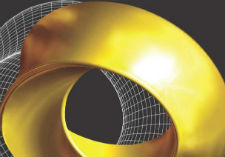AP Calculus AB

This course is intended for students who have a thorough knowledge of analytical geometry and elementary functions in addition to algebra, geometry, and trigonometry. It is a course in single-variable calculus that includes techniques and applications of the derivative, techniques and applications of the definite integral, and the Fundamental Theorem of Calculus. It is equivalent to at least a semester of calculus at most colleges and universities, perhaps to a year of calculus at some. Algebraic, numerical, and graphical representations are emphasized throughout the course.The course includes the application of limits, differentiation and integration. Some techniques of integration and indeterminate forms for limits are also covered. Throughout the course, an emphasis is placed on symbolic, graphical, and numeric representations, as well as on clear communication of mathematical thinking. Students successfully completing this course are prepared to take the Calculus AB AP Exam which requires use of a graphing calculator .

Major Units:

• Limits and Continuity – graphical, numerical, and verbal descriptions of a limit; graphical, numerical, algebraic and verbal evaluation of limits; indeterminate forms; three-part definition of continuity at a point; types of discontinuity; redefining functions to be continuous; piecewise-defined and greatest integer functions; intermediate value and extreme value theorems
• The Derivative: derivative as a limit and rate of change; differentiability; differentiation using the limit definition and through algebraic and graphical shortcuts; the product, quotient and chain rules; tangent lines; derivatives of transcendental and piecewise-defined functions; derivatives of inverse functions; review of conic sections and other planar curves; implicit differentiation; related rates of change
• Derivative Applicatioon: first derivative applications, second derivative applications, graphical construction and analysis, the Mean Value Theorem,
• Anti-Derivatives and Indefinite Integrals: slope fields; differential equations with separation of variables; anti-derivatives with initial conditions; anti-derivatives using the power rule and substitution; anti-derivatives of basic transcendental functions
• The Definite Integral and Applications: Riemann sums; ordering estimations of sums; evaluating definite integrals; areas in the plane; solids of revolution and with other cross-sections using the washers and shells methods; applications to population, finance/economics, fluid dynamics, thermodynamics; the First and Second Fundamental Theorems of Calculus